Edge Detection Operators

Operators are used to detect edges present in an image. In general, operator sizes depend on the sizes of the edges and the resolution of the image. This is defined as the "Resolution problem."

• Calculated points are in between the pixels.
 Roberts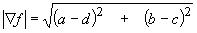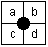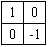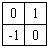Forsen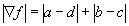Ejiri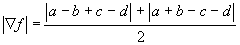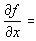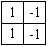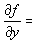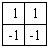• Calculated points are in the pixels.
 Rosenfeld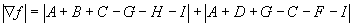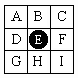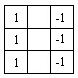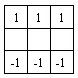Sobel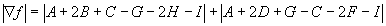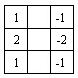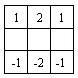• To locate an edge where the magnitude of the gradient vector is larger than the threshold value.
 Original Gradient vector field Detected edge

• Laplacian Operators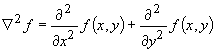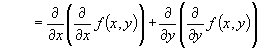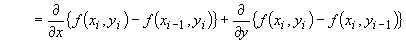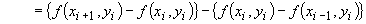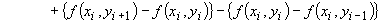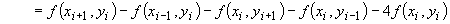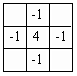To locate an edge where Laplacian has a zero crossing.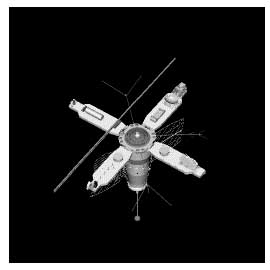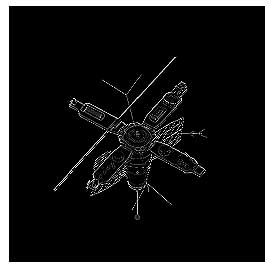Original Laplacian operator

• Operator based on edge model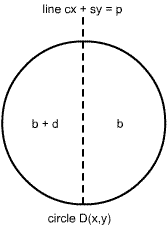Graphic of edge model Graphic of "cx + sy = p"

 Step edge model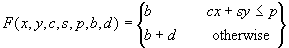Problem specification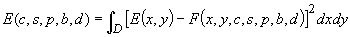It is necessary to minimize E(c,s,p,b,d) to obtain c,s,p,b, and d values Remarks Optimal edge is obtained Computation cost is high

At each pixel, calculate the response of the mask. The direction which gives the maximum response defines the direction and strength of the edge.

 Prewitt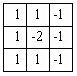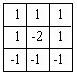Kirsch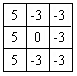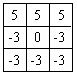Remark: It is necessary to keep the average power of the mask zero
• Laplacian Gaussian operator
• Laplacian may enhance noise
• Gaussian may smooth noise
 Laplacian Gaussian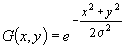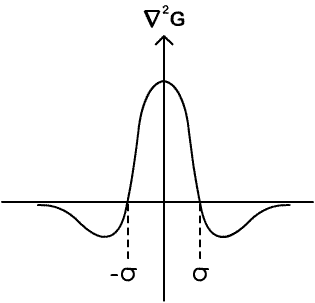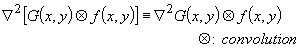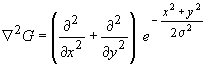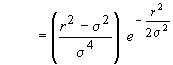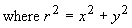• Canny operator

Optimized edge detector

Condition

• Probability to detect real edge has to be high and probability to detect false edge has to be low.
• The location detected is close to the real edge location
• Only one response for a real edge

The optimized edge detector is approximated by the first derivative of Gaussian.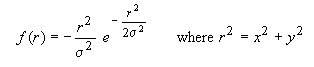• Edge Point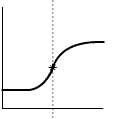Profile of an image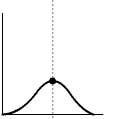First derivative: point of the maximum value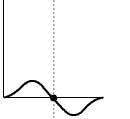Second Derivative: point where the value changes sign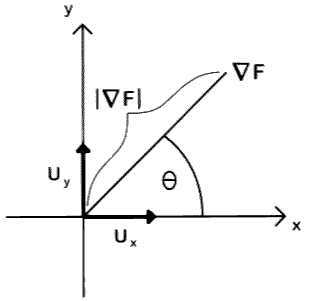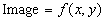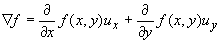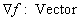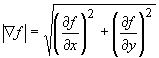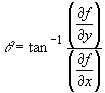• Example of a gradient vector field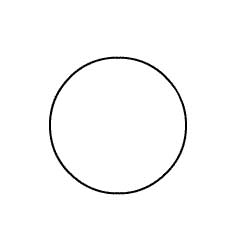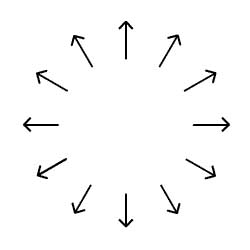Circle Gradient vector field of a circle
• How to calculate gradients for digital images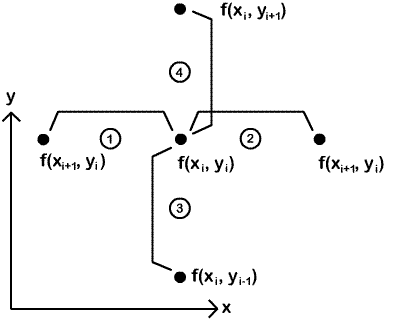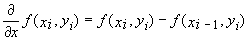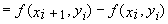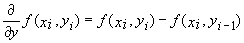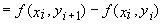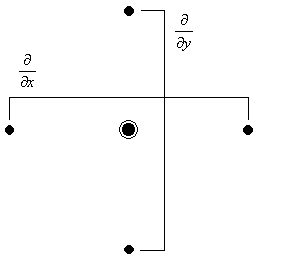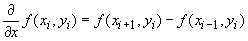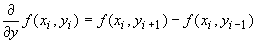A smooth edge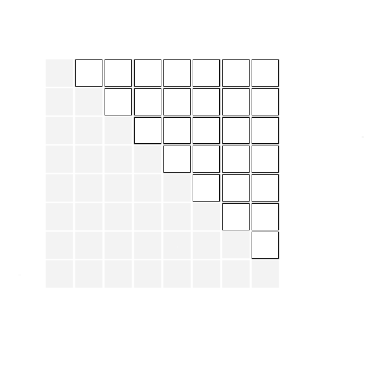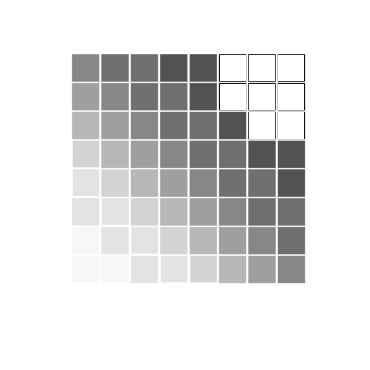On this fixed 3x3 (or any fixed pxq) neighborhood centered on any pixel position (m,n), the differences in the various directions are

f(x,y)/y = [f(m,n+1) - f(m,n-1)]/2 (3.10a)

f(x,y)/x = [f(m+1,n) - f(m-1,n)]/2 (3.10b)

f(x,y)/u = [f(m+1,n+1) - f(m-1,n-1)]/2 (3.10c)

f(x,y)/v = [f(m-1,n+1) - f(m+1,n-1)]/2 (3.10d)

where u is in the direction along the line y = x and v is the direction along the line y = -x.

Masks that produce these differences under convolution (ignoring the 1/2 factor) are

0 0 0 0 -1 0 -1 0 0 0 0 1

-1 0 1 0 0 0 0 0 0 0 0 0

0 0 0 0 1 0 0 0 1 -1 0 0

More effective differencing masks average more than a single difference of pixels to mitigate errors due to a noisy pixel. These Prewitt operators are

-1 0 1 -1 -1 -1-1 -1 0 0 1 1

-1 0 1 0 0 0 -1 0 1 -1 0 1

-1 0 1 1 1 1 0 1 1 -1 -1 0

More effective yet are the Sobel operators that weight key differencing pixels more, which are

-1 0 1 -1 -2 -1 -2 -1 0 0 1 2

-2 0 2 0 0 0 -1 0 1 -1 0 1

-1 0 1 1 2 1 0 1 2 -2 -1 0

Note that the sum of the entries in each differencing mask is zero. This means that if a neighborhood consists of pixels of constant value a, then the new pixel has value

gc = (j=0,pq-1) hjfj = a(j=0,pq-1) hj = (a)(0) = 0 (3.11)

Thus pixels with near constant neighborhood values are changed to near black (near 0). If the average of the pixels in the positive direction is greater than that in the negative direction from the center, then the new pixel value gc will be brighter and contrast against the darker background. If the average pixel value in the negative direction is greater than that in the positive direction, then the new pixel value computed will be negative, and will be truncated to zero and so will be black (0). For L levels of grayscale, all computed pixel values are bounded by 0 and L-1 (usually 0 and 255). Thus the difference (edge detecting) operators work in only one direction.

After convolution with all four difference operators, the edges will be light lines on a dark background in four different images. These can be thresholded to bilevel images of black and white and then combined via use of the maximum at each pixel.

Laplacian Masks. The Laplacian sums the squares of the second derivatives via

2f(x,y) = 2f(x,y)/x2 + 2f(x,y)/y2 (3.12)

Recalling that the second derivative can be approximated by the difference of two derivatives at two adjacent points, the second derivatives become

2f(x,y)/x2 = {[f(m+1,n) - f(m,n)]/ - [f(m,n) - f(m-1,n)]/} / =

{[f(m+1,n) - 2f(m,n)] + f(m-1,n)} / 2 (3.13)

2f(x,y)/y2 = {[f(m,n+1) - f(m,n)]/ - [f(m,n) - f(m,n-1)]/} / =

{[f(m,n+1) - 2f(m,n)] + f(m,n-1)} / 2 (3.14)

The 3x3 Laplacian mask is therefore the sum of the two second differencing masks, which is

0 1 0 0 0 0 0 1 0

0 -2 0 + 1 -2 1 = 1 -4 1 (3.15a)

0 1 0 0 0 0 0 1 0

when is ignored ( = 1 = 2). The negative Laplacian is used mostly (see Fig. 3.13 and discussion).

0 1 0 0 -1 0

(-1) 1 -4 1 = -1 4 -1 (3.15b)

0 1 0 0 -1 0

Note, for example, that both the negative and positive Laplacian processes produce an output gc near zero (very dark) when all pixels on the matching "plus-shaped" neighborhood are approximately the same (the mask entries sum to zero). On the other hand, the negative Laplacian produces a positive output only when the central pixel value is greater than the average of the others. If the central pixel value is less than the average of the others, then the negative result is truncated to zero (black).

Hedged Laplacians. Figure 3.10 uses the negative 3x3 Laplacian given in Equation (3.15b) on the original "Shuttle", while Figure 3.11 shows the effect of using the hedged Laplacian mask of Equation (3.16).

-1 -1 -1 0 0 0 -1 -1 -1

-1 9 -1 = 0 1 0 + -1 8 -1 (3.16)

-1 -1 -1 0 0 0 -1 -1 -1

Figure 3.10. Laplacian on "shuttle" Figure 3.11. Hedged Laplacian on "shuttle"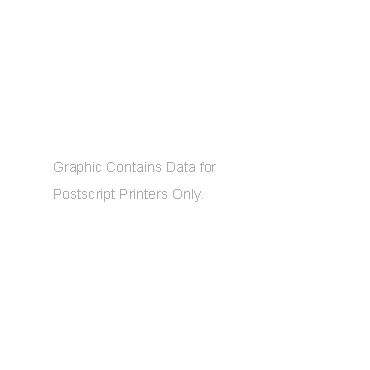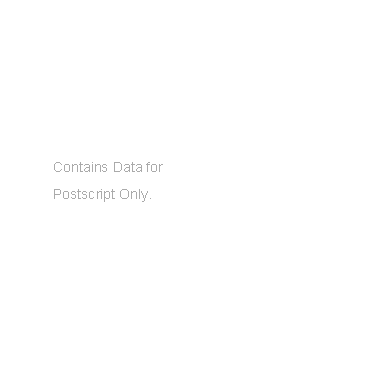Hedged Laplacians can be considered to add the negative Laplacian processed image back onto the original image. This is actually the subtraction of the Laplacian from the original image, which is a powerful technique as shown in Figure 3.11. Note that the sum of the weights in the hedged Laplacian is equal to unity, so the average gray level will remain the same as in the original image. While the image in Figure 3.11 is sharp, the small differences due to random effects (noise) are exaggerated (a disadvantage of Laplacian and other differencing methods). A hedged Laplacian mask that sharpens less severely is

0 -1 0 0 0 0 0 -1 0

-1 5 -1 = 0 1 0 + -1 4 -1 (3.17a)

0 -1 0 0 0 0 0 -1 0

Other interesting hedged Laplacians are

-1 -2 -1 0 0 0 -1 -2 -1

-2 13 -2 = 0 1 0 + -2 12 -2 (3.17b)

-1 -2 -1 0 0 0 -1 -2 -1

-1 -1 -1 -1 -1

-1 -1 -2 -1 -1

-1 -2 29 -2 -1 = ? (3.17c)

-1 -1 -2 -1 -1

-1 -1 -1 -1 -1

Figure 3.12. Edge cross-sections Figure 3.13. Subtracting the Laplacian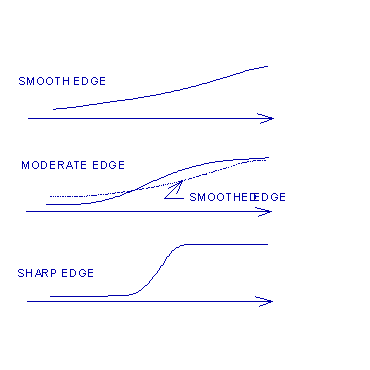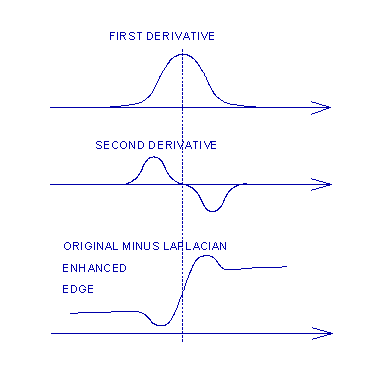Edge Enhancement. A cross-section of an image shows edges as rises in Figure 3.12 (here the dark side is on the left and the light side is on the right). Figure 3.13 shows the first and second derivatives of the moderate edge and the effect of adding the original to the (negative) Laplacian, which is actually subtracting the Laplacian from the original (see the bottom graph). This is analogous to unsharp masking, where the smoothed edges (see the center graph of Figure 3.12) are subtracted from the original edges to sharpen them, which yields a result similar to the bottom graph of Figure 3.13.

A variation on the Laplacian processing is to check the value of each Laplacian against a fixed threshold T. If the Laplacian is too large (larger than the threshold), then it is likely to be erroneous due to noise and should be suppressed. In this case the neighborhood average is taken to be the new pixel value gc. On the other hand, if the Laplacian is low (lower than the threshold), then the new pixel value gc is enhanced by adding a proportion of the Laplacian to it. The algorithm is

if 2f(m,n) > T then gc = (1/r)(k=1,r) fk

else gc = f(m,n) + ß2f(m,n)

The disadvantages of Laplacian operators are that they give no direction for the edge, they produce double edges, and they are very sensitive to noise. Derivatives and differences are sensitive to noise and second differences are even more sensitive in that small errors in the input data can cause large errors in the output data. Noise should be reduced before edge detection is performed.

The gradient can be used in situations where it is important to know the direction of the edge or line segment. The gradient and direction can be found per

(f(x,y)/x,f(x,y)/y) = (f(m+1,n) - f(m-1,n), f(m,n+1) - f(m,n-1)) (3.18)

= atan(f(x,y)/y / f(x,y)/x) (3.19)

3.5 Line Drawings

Line Detection. Lines are extended edges. A line drawing can be developed from an image {f(m,n)} by convolving an edge detector such as the Laplacian or the four Sobel operators. These yield light lines on a black background, so we invert by putting g(m,n) = 255 - f(m,n) to achieve black lines on a white background. We can also use a threshold to transform the image to black and white only.

A problem is that the lines are too thick (edge detection thickens the lines). Other problems include broken lines and noise. It is useful to remove noise before processing to detect lines, but this should not be done by smoothing via averaging because this spreads and weakens the edges. The thinning of lines after line detection is often necessary, as is the removal of spurs sticking out from the lines at various angles. The processes of thinning lines and trimming spurs from them is covered in Chapter 10.

Line detection is a type of edge detection. Consider a horizontal line segment in an image. If the pxq neighborhood straddles a horizontal line, for example, that line could be detected if the pixels along the center row have a higher average than those of the other rows. Thus

-1 -1 -1 -1 -1 -1 -1 -1

2 2 2 2 2 2 2 2

-1 -1 -1 -1 -1 -1 -1 -1

are horizontal line detectors. The larger mask is more immune to noise. Similarly detectors of vertical, -45 and 45 lines are

-1 2 -1 -1 2 -1 -1 -1 2 -1 -1 -1 -1 4 2 -1 -1 4 -1 -1 -1 -1

-1 2 -1 -1 2 -1 -1 2 -1 -1 -1 -1 4 -1 -1 2 -1 -1 4 -1 -1 -1

-1 2 -1 -1 2 -1 2 -1 -1 -1 -1 4 -1 -1 -1 -1 2 -1 -1 4 -1 -1

-1 2 -1 -1 4 -1 -1 -1 -1 -1 -1 4 -1

-1 2 -1 4 -1 -1 -1 -1 -1 -1 -1 -1 4

We can now answer an important question: is it possible to detect lines in all directions with a single mask? Upon adding the 3x3 horizontal and vertical line detector masks first to obtain a horizontal-vertical line detector, and then adding the 3x3 diagonal line detector masks second to obtain a diagonal line detector, we obtain the two sums

-2 1 -2 1 -2 1

1 4 1 -2 4 -2

-2 1 -2 1 -2 1

The sum of these two masks yields a mask that detects edges in the horizontal, vertical, -45 and 45 diagonal directions, which is

-2 1 -2 1 -2 1 -1 -1 -1

1 4 1 + -2 4 -2 = -1 8 -1 (3.20)

-2 1 -2 1 -2 1 -1 -1 -1

Thus we have proved that the Laplacian mask on the righthand side of Equation (3-20) detects edges in all directions (quod erat demonstrandum).

Line Drawings with XV. Figure 3.14 displays a line drawing of the "Shuttle" image obtained by first applying the Laplacian of Equation (3-20) and then inverting via the transformation

g(m,n) = 255 - f(m,n) (3.21)

Figure 3.15 shows the line drawing obtained by thresholding to black and white.

To invert, we ran XV (characters typed by user are in boldface) per

images2> XV in_filename.pgm

Then we selected the contol window by: i) right-clicking inside the image to get the control window; ii) selecting Windows in the center of the menu bar; iii) selecting Color Edit; and iv) changing the intensity transformation in the small box at the bottom center of the large Color Edit window. We inverted this linear graph from y = x to y = 255 - x by clicking on the small square nodes, holding the button down and dragging the lower lefthand corner to the upper lefthand corner and the upper righthand corner to the lower righthand corner. Next, we moved the squares to form a threshold transformation with g(m,n) = 0 for f(m,n) < 128 and g(m,n) = 255 for f(m,n) 128.

Figure 3.14. Inverted edges of "shuttle" Figure 3.15 Thresholded inverted "shuttle"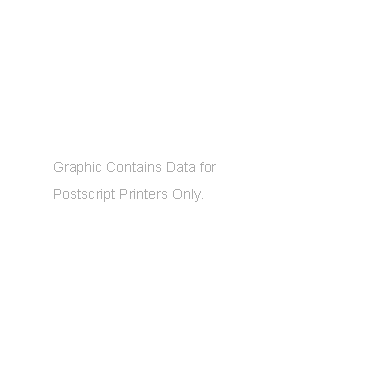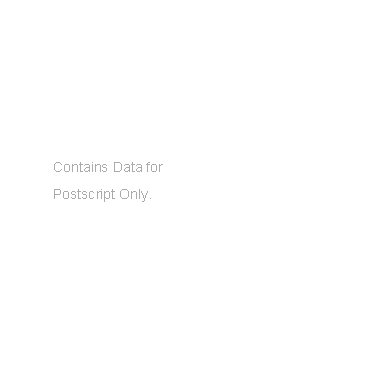3.6 An Algorithm for Mask Convolution

In this algorithm, the mask of weights that contains p rows and q columns will be represented by {h(i,j): 0 i p-1, 0 j q-1}. This algorithm will process a pxq neighborhood of each pixel in the image {f(m,n): 0 m M-1, 0 n N-1}. It merely copies the pixels whose pxq neighborhood overlaps the image, that is, is not completely in the image.

Figure 3.16. Processing a row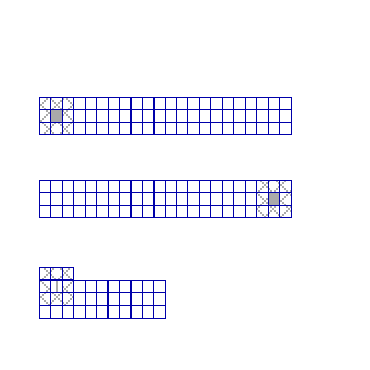The first pixel in the upper lefthand corner to be processed will be located at the point (p/2,q/2). Recall that p and q are odd integers, so p/2 and q/2 are truncated to be the central pixel position (m,n) = (p/2,q/2) in the pxq neighborhood. The algorithm works on a strip of p rows, of which p/2 is the row to be processed (see Figure 3.16). Considering pxq = 3x3, the point (p/2,q/2) = (1,1) is the first pixel to be processed on the strip of rows 0, 1 and 2 with columns 0, 1 and 2 being the first 3x3 neighborhood. The last pixel in this row is at (p/2,N-1) but it can not be processed because it does not have a complete neighborhood in the image. Thus the last pixel in row p/2 to be processed is (p/2,N-1-q/2).

On a first reading of the algorithm given below, let p = 3 and q = 3. Figure 3.16 presents a view of a strip of rows being processed. The mask overlaps the image at the first image pixel (top left) and so we do not process the top row of pixels. Similarly, we do not process the first column nor the last, nor the last row. The greatest integer for pxq = 3x3, is p/2 = q/2 = 1. In the first strip shown, we start the mask over a neighborhood (nbhd) in the image, and process the neighborhood to obtain a new center pixel. Then the mask moves to the right and processes the next neighborhood, and so forth, until it reaches the last one for which the mask fits inside the image strip. We write the first pixel and last pixel in the strip directly to the output array without processing. Similarly, we write the first row and last row of the image to the output array. Appendix 3b lists a C program for this.

call GetStrip(); /Procedure gets first p rows, 0,...,p-1, from/

for m = 0 to (p/2)-1 do /input file and places them in array f[m][n]/

for n = 0 to N-1 do /Read and write first p/2 rows to output array/

g[m][n] f[m][n]; /g[m][n] for writing directly to output file/

RowCount p/2; /First row to be processed/

do /Loop over all strips of p rows/

ColCount 0; /First q/2 columns can not be processed/

for n = 0 to (q/2)-1 do /but are copied directly to output array/

g[RowCount][n] f[RowCount][n]; /Write unprocessed pixels in row to output array/

do /Loop over all processable columns in strip/

PixelSum 0; /Initialize convolution sum to zero/

for mrow = 0 to p-1 do /For all pixels (m,n) in current/

for ncol = Colcount to Colcount+q-1 do /neighborhoo and all mask entries/

deltacol ncol - ColCount; /(mask columns always go from 0 to p-1)/

PixelSum PixelSum + mask[mrow][deltacol]*f[mrow][ncol]; /do convolution/

NewPixel integer(PixelSum); /Put real pixel value into integer variable/

if (NewPixel > 255) then NewPixel 255; /New pixel must be between 0 and 255/

if (NewPixel < 0) then NewPixel 0 ; /for grayscale processing/

g[RowCount][Colcount+q/2] NewPixel; /Put processed value into output array/

ColCount ColCount + 1; /Increment column to be processed next/

while ColCount N-(q/2)-1; /& process until nbhd goes outside of image/

for n = N-(p/2) to N-1 do /Copy last (q/2) cols. of row to output array/

g[RowCount][n] f[RowCount][n]; /for current row being processed/

RowCount RowCount + 1; /Increment count of row for processing next/

while GetStrip() != -1; /Get next strip of rows (move down 1 row)/

/and place it them in array f[m][n]/

for m = M-(p/2) to M-1 do /Read/write last p/2 rows directly to output/

for n = 0 to N-1 do /g[m][n] for writing to output file/

g[m][n] f[m][n];

On the first call the procedure GetStrip() reads the first p rows (indexed as 0,...,p-1) into the array variable f[m][n] as the first strip. The first p/2 rows are not processed because no complete neighborhoods exist for them and so they are written directly to the output array. The row count is set at p/2, which is the first row to be processed. The first (q/2) pixels in that row are not processed (no complete neighborhood exists) and so are copied directly into the output array. Then the column count is set at zero and the first q columns are used as the first neighborhood. Mask convolution processes it to obtain the new output pixel g[p/2][q/2]. After that, the column count is incremented by one to move the mask to the right one pixel and the mask convolution is done again, and so forth.

When the processing of the current row (designated by RowCount) is completed (all pixels in the row have been processed except the first 0,...,(q/2)-1 and the last N-(q/2) columns), then these last (q/2) pixels are copied directly to the output array (the first q/2 pixels in this row wre previously copied). Then a new strip of p rows that consists of rows Rowcount,...,Rowcount+p-1 is placed in f[m][n] for m = 0,...,p-1 by GetStrip() and is processed the same way. When the procedure GetStrip() gets the last p rows M-p,...,M-(p/2)-1, the returned value is still 1 as in all previous cases. The next time, however, it returns -1 to indicate that there is no complete strip to process (no complete neighborhoods exist for the pixels in the remaining rows) and the process terminates. The last p/2 rows are copied into the output array g[m][n] directly. Another procedure can be used to write the output row to the output image file during processing, or the entire array {g[m][n]) can be written to the file at the end. Appendix 3b presents C source code for mask convolution.

3.7 UNIX System Calls to XV

It is convenient to make a system call to XV from the image processing program to display an image on the screen. A system call interacts with the operating system (a UNIX type) just as if the programmer were typing from the command line. The commands must be written into a buffer and given to the system call instruction. As before, X-windows must be running.

We prefer to first display the original image with a system call to XV and then display the processed image with another system call. We use the poll parameter so that we may process the image again, write the processed image to the output image file and display the processed image in the same processed image display window as before.

A separate subroutine (procedure, or function) can be written to make the system calls. We call this procedure Display() and call it from the main routine in the program at the end of a loop that processes the image. Then the user can exit from the loop or select parameters and so forth and process the image again. When the repeat processing is done and written to the output file, then that image is displayed by XV. Our high level main procedure contains the subprocedures in the order given below.

OpenFiles(); /Opens input and output image files/

do /Start of do-while loop for repeat processing/

ApplyMask(); /Calls GetStrip(), does convolution on image/

/and writes processed data to output array/

Display(); /Calls XV to display images/

key GetUserInfo(); /User inputs key to stop or process again/

while key != 'x'; /End of do-while loop, 'x' exits loop/

CloseFiles(); /Write data to output files, close files/

The C code for the Display() procedure uses the string print function sprintf() to write the command to be given to the system call into a buffer. The first buffer is for the command that copies the output file (outfile) to a new output file (display_file) to be used in the display. It is called buffer1. This is accomplished via

sprintf(buffer1, " %s %s %s ", " cp ", outfile, display_file);

This writes three string variables into buffer1. The first is the string "cp" to give the UNIX command to copy a file. The second is the name of the processed (source) output file outfile and the third is the name display_file of the destination file to use for displaying the image.

The buffer that holds the command to call XV to display the image is buffer2. We next use

sprintf(buffer2, " %s %s %s ", " xv -poll -geometry +10-50 ",display_file);

This writes four string variables in a buffer (buffer2). The first is the command xv that causes the operating system to run the XV program. The second is the parameter that tells XV to poll to see if the image file has changed and to update the display if it has. The third gives the location on the screen of the image, which in this case is at 10 pixels from the left and 50 from the bottom of the screen. The fourth is the name of the file to display, which here is display_file.

To actually execute the commands stored in buffer1 and buffer2 we use the system() function per

system(buffer1); /Execute file copy command in buffer1/

system("sleep 2"); /Wait for file copy to be completed/

system(buffer2); /Buffer2 comand calls XV to display image/

These system commands can be put in loops or branches and so forth, so the programmer can be quite creative in the use of XV to display the images. We use bufferA and bufferB to hold similar commands for display the original image, except that we do not use the "-poll" parameter (the original stays the same throughout and only the reprocessed output image changes). Appendix 3a provides the C source code for Diplay().

3.8 Exercises

3.1 Is it possible to construct a 5x5 mask that detects a weak (smooth) edge that is dark on the left side and light on the right side, but that doesn't use the middle column of the mask (it consists of zeros)? If so, explain how it works.

3.2 Develop a mask convolution process whereby a pixel is smoothed if all pixels in its neighborhood are close in graylevel to its graylevel but is sharpened if if a majority of the neighborhood pixels differ significantly. Use neighborhoods of differences between the pixels and the origin (center) pixel.

3.3 Discuss the effect of unsharp masking when ß > 1 and the smoothing mask to be subtracted is multiplied by = ß - 1.

3.4 What is the result of adding a sharpening and a smoothing mask to obtain a mask whose entries sum to 1?

3.5 Develop a 5x5 Laplacian type mask by adding line detector masks for the horizontal, vertical and ±45 directions.

3.6 Complete Equation (3.17c). Explain the effects of each of the two parts.

3.7 Run XV on shuttle.pgm and invert it by transforming the histogram.

3.8 Use XV to threshold the inverted image of Exercise 3.6 to convert it to black and white.

3.9 Write a simple program in C that performs mask convolution (see Appendix 3b).

3.10 Perform unsharp masking on "Lena" to sharpen it by entering a single mask into the program of Exercise 3.9. What effect does ß have (try a higher and lower value than ß = 2)? What effect does the degree of smoothing have? Experiment with and different degrees of smoothing.

3.11 Incorporate the C function of Appendix 3a into the program of Exercise 3.9 so the original, processed, and all reprocessed images will display on the screen.

Appendix 3a - A Display Function in C for Use with XV

void Display()

{

/***Part 1. Copy original image "infile" to file "Display_File1" and display on first time***/

if (first_time == 1)

{ /*-----Command to copy original image file "infile" to file "Display_File1"-----*/

sprintf(bufferA, " %s %s %s " , " cp " , infile, Display_File1);

/*-----Give system command in "bufferA" to system to execute copy-------------*/

system(bufferA);

/*-----Wait for image file to be completely copied------------------------------------*/

system("sleep 2");

/*-----Command to display image in "Display_File1" with XV at (2,2)------------*/

sprintf(bufferB, " %s %s %s " , " xv -geometry +2-2" , Display_File1,"&");

/*-----Display original image in "Display_File1" with XV---------------------------*/

system(bufferB);

}

/*========================================================================*/

/***Part 2. Copy processed image "outfile" to file "Display_File2" and display on any call***/

/*-----Command to copy processed file "outfile" to file "Display_File2"-----------*/

sprintf(buffer1, " %s %s %s " , " cp ", outfile, Display_File2);

/*-----Give system command in "buffer2" to system to execute copy----------------*/

system(buffer1);

/*-----Wait for image file to be completely copied---------------------------------------*/

system("sleep 2");

if (first_time == 1)

{ /*-----Command to display image in "Display_File2" with XV at (200,200)------*/

sprintf(buffer2, " %s %s %s ", " xv -poll -geometry +200-200 " , Display_File2,"&");

/*-----Display processed image in "Display_File2" with XV------------------------*/

system(buffer2);

/*-----Turn off first_time so as to not call XV further--------------------------------*/

first_time = 0;

/*-----The polling feature updates changed processed files automatically----------*/

}

printf(" Move images to desired location for viewing and comparison!\n");

return;

} /* End of Display() function */

Notes:

i) infile and outfile are names of files given by the user to read the input image file and write the output image file, respectively. They are declared in main via

char infile;

char static outfile;

ii) Display_File1 and Display_File2 are names of the files to which the original image file infile and outfile are copied, respectively. They are declared in main via

char Display_File1 = "Image_Before";

char Display_File2 = "Image_After";

iii) the buffers are strings that are declared in main via

char bufferA, bufferB, buffer1, buffer2;

Obviously, a single buffer could be used to store each command in a sequential fashion before making a system call, but we have taken the pedagogical approach.

iii) first_time is an integer variable declared in main and set to 1. After an image has been processed, the function Display() is called to display the original and the processed images in two separate windows. Then first_time is turned off, that is, set to 0 so that no further processing of the original image will be displayed by a system call. However, any reprocessing of the image and call of Display() causes the new reprocessed image to be copied to Display_File2. The poll parameter causes the new reprocessed image in Display_File2 to be written to the display in the window over the previous processed image.

Appendix 3b - A Mask Convolution Program in C

/*+++++++++++++++++++++++++++<M D I P>+++++++++++++++++++++++++++++++++*/

/* */

/* >>>MDIP 3.1 - MASK DIGITAL IMAGE PROCESSING Program<<< */

/* Linux Version -- Gnu C++ Compiler */

/* (also UNIX Version with C++ Compiler) */

/* */

/* This program processes an input image with a user */

/* supplied (user input) convolution mask, writes out result. */

/* */

/*--------------------------------------------------------------------- */

/* It makes calls to XV (Xview) to display original and */

/* processed images on screen. Reprocessed images are updated */

/* on the screen. */

/*=====================================================================*/

/* Version 1.0.9.99 */

/*--------------------------------------------------------------------- */

/* This program processes a *.pgm image of raw data of size up */

/* to 1280 (pixels wide) by M (pixels high) where M can be any */

/* reasonable value, say 768, 1024, 1280, 4096, etc. The user */

/* provides the input image file name and an image output file */

/* where the results will be written. The output file is written */

/* as a stream of characters where each is a gray scale value */

/* of from 0 to 255. The output file is a *.pgm file (raw data). */

/* ---------------------------------------------- */

/* The mask height and width are selected so that each is one */

/* of {3,5,7,9,11,13,15,17,19,21} independently of the other. */

/* The user must input the mask. */

/* */

/*=====================================================================*/

/*------------------------P R O G R A M-------------------------------- */

/*=====================================================================*/

/* */

/* main(); */

/* instruct(); displays instructions if selected */

/* openfiles(); opens input & output image files */

/* getstrip(); reads strip of rows from input image file */

/* convolve(); performs convolution of mask and row strip */

/* writefile(); writes processed row to output image file */

/* lastrows(); writes last rows (unprocessed) to output file */

/* closefiles(); closes input and processed output image files */

/* display(); displays original and processed image via XV */

/*--------------------------------------------------------------------- */

# include <stdio.h>

# include <math.h>

# include <stdlib.h>

/*--------------------------------------------------------------------- */

void openfiles(void);

void instruct(void);

void display(void);

/*--------------------------------------------------------------------- */

int fin;

int gout;

int MRows, NCols; /*indices for rows and cols*/

int p, q; /*height/width of pxq mask*/

int rowcount; /*count of row being processed*/

FILE *infptr, *outfptr; /*input/output file pointers*/

char infile;

char static outfile; /*names of input/output image files*/

char key; /*key to select instructions*/

char display_File1[] = "Original_Image";

char display_File2[] = "Processed_Image";

int first_time;

float factor; /*multiplier of convolution mask*/

/*---------------------------------------------------------------------- */

/*-----------------------------MAIN------------------------------------- */

/*---------------------------------------------------------------------- */

main()

{ void closefiles();

char changekey, stopkey; /*key to process another image or stop*/

do

if (key == 'i') instruct();

first_time = 1;

do

{ /*---------------(Open Input & Output Image Files)---------------- */

openfiles();

/*----------------(Get Mask Entries & Multiplier)----------------- */

/*---------------(Do Convolution on Image with Mask)-------------- */

/*---------------(Display Image with XV Program)------------------ */

display();

/*---------------(Select to Change this Image or Not)------------- */

do

{ printf("\n Process this image again with new parameters (y/n): ?");

scanf("%1s",&changekey);

} while ((changekey!='y') && (changekey!='Y') &&

(changekey!='n') && (changekey!='y'));

} while ((changekey=='y') || (changekey=='Y'));

/*------------------(Close Any Open Image Files)-------------------- */

closefiles();

/*----------------(Select Stop or Process Another Image)------------ */

do

{ printf("\n Enter <s> to stop or <i> to process another image ");

scanf("%1s",&stopkey);

} while ((stopkey != 's') && (stopkey != 'S') &&

(stopkey != 'i') && (stopkey != 'I'));

/*------------------------------------------------------------------ */

} while ((stopkey != 's') && (stopkey != 'S'));

printf("\n Bye! Bye!\n");

return;

} /*end main()*/

/*---------------------------------------------------------------------- */

/*---------------------------------------------------------------------- */

{ int i;

for (i=0;i<16;i++) printf(" +\n");

printf(" MDIP Ver. 1.2 - Convolution MASK DIGITAL IMAGE PROCESSING\n");

printf(" by Prof. Carl G. Looney\n");

printf(" Computer Science Department/171\n");

printf(" Reno, NV 89557\n");

printf(" looney@cs.unr.edu\n");

printf(" Updated: 22Sep97\n");

for (i=0;i<4;i++) printf(" +\n");

do

{ printf("\n Enter <i> for instructions or <c> to continue: ");

scanf("%1s",&key);

} while ((key != 'i') && (key != 'c'));

return;

/*---------------------------------------------------------------------- */

/*---------------------------INSTRUCT----------------------------------- */

/*---------------------------------------------------------------------- */

void instruct(void)

{ printf("\n\n"); printf("\n\n"); printf("\n\n");

printf(" >> I N S T R U C T I O N S <<"); printf("\n\n");

printf(" This program processes RAW (packed) DATA *.PGM image files\n");

printf(" (from XView) for 0 - 255 GRAY LEVELS. The image processing is\n");

printf(" done via convolution with a pxq MASK that is entered by the\n");

printf(" user, where p,q are each in {3, 5, 7, 9, 11, 13}. The image\n");

printf(" file header must have 4 lines, and the third line must give\n");

printf(" N = number of columns and M = number of rows in the image.\n");

printf("------------------------------------------------------------\n\n");

printf(" <P5> (Line 1: P5 => raw packed bytes, P2 => ascii codes)\n");

printf(" <# ....> (Line 2: a comment)\n");

printf(" <640 480> (Line 3: no. columns and no. rows)\n");

printf(" <255> (Line 4: max. no. of gray levels)\n");

printf("-------------------------------------------------------------\n\n");

printf(" The input .PGM file is processed and the results are written\n");

printf(" to the .PGM output file. The mask MULTIPLIER is composed of\n");

printf(" integer inputs for NUMERATOR and DENOMINATOR.\n\n");

printf(" Please write the mask and multiplier parts on paper and enter.\n");

printf("--------------------------------------------------------------\n\n");

printf(" Use a text editor if you need to fix the 4 line header on the\n");

printf(" image file to (e.g., XV may put in an extra comment line!).\n\n");

return;

}/*end instruct()*/

/*---------------------------------------------------------------------- */

/*--------------------------OPENFILES----------------------------------- */

/*---------------------------------------------------------------------- */

void openfiles(void)

if (first_time == 1)

{ printf("\n OPEN an image file\n");

printf("~~~~~~~~~~~~~~~~~~~~~~~~~~~~~~~~~~~~~~~~~~~~~~~~~~~~~~~~~~~~~~~\n");

printf(" Enter name of *.pgm INPUT image file: ? ");

scanf("%s",&infile);

printf(" Enter name of *.pgm OUTPUT image file: ? ");

scanf("%s",&outfile);

}

if ((infptr = fopen(infile, "r")) == NULL)

{ printf(" Can NOT open input image file: <%s>\n",infile);

printf(" Exiting program..... "); exit(1);

}

else printf(" Input file <%s> opened sucessfully\n\n",infile);

if ((outfptr = fopen(outfile,"w")) == NULL)

{ printf(" Can NOT open output image file <%s>\n\n",outfile);

printf(" Exiting program....."); exit(1);

}

else printf(" Output file <%s> is opened sucessfully\n\n",outfile);

return;

}/*end openfiles()*/

/*---------------------------------------------------------------------- */

/*---------------------------------------------------------------------- */

{ char redo; /*key to redo the input mask*/

int i, j;

float numer, denom;

printf(".......+..........+............+...........+............+.......\n");

printf(" Image is to be processed by:\n");

printf(" -- it requires INTEGER entries\n\n");

do

{ do

{ printf("\n Enter height of convolution mask (3,5,7,9,11,13,15,17,19,21): ?");

scanf("%d",&p);

} while ((p!=3) && (p!=5) && (p!=7) && (p!= 9) && (p!=11) && (p!=13)

&& (p!=15) && (p!=17) && (p!=19) && (p!=21));

do

{ printf(" Enter width of convolution mask (3,5,7,9,11,13,15,17,19,21): ?");

scanf("%d",&q); printf("\n");

} while ((q!=3) && (q!=5) && (q!=7) && (q!=9) && (q!=11) && (p!=13)

&& (q!=15) && (q!=17) && (q!=19) && (q!=21));

printf(" Enter mask multiplier NUMERATOR (integer): ?");

scanf("%f",&numer); printf("\n");

printf(" Enter mask multiplier DENOMINATOR (integer): ?");

scanf("%f",&denom); printf("\n\n");

factor = numer/denom;

for (i=0;i<p;i++)

{ printf(" Row (INTEGERS) %d::\n",i); printf(" __________________\n");

for (j=0;j<q;j++)

}

printf("\n");

}

printf(" User's Convolution Mask (multiplier = %f):\n",factor);

for (i=0;i<p;i++)

{ for (j=0;j<q;j++) printf(" %f ",mask[i][j]);

printf("\n");

}

printf("+++++++++++++++++++++++++++++++++++++++++++++++++++++++++++\n\n");

printf(" Hit <a> to accept given mask or <r> to redo it: ?");

scanf("%1s",&redo); printf("\n\n");

} while (redo == 'r');

/*---------------------------------------------------------------------- */

/*---------------------------------------------------------------------- */

{

void getstrip(void);

void convolve(void);

void writefile(void);

void lastrows(void);

void closefiles(void);

int Mminusphalf, Nminusqhalf, Mend;

int pm1, phalf, phalfp1;

pm1 = p - 1; phalf = p/2; phalfp1 = phalf + 1;

rowcount = 0;

Mend = MRows - phalf;

getchar();

do

{ /*------------[Read Strip of p Image Rows to Process]--------------- */

getstrip();

/*-----------------[Convolve Row Strip with Mask]------------------- */

convolve();

/*--------------[Write Processed Row to Output File]---------------- */

writefile();

rowcount++;

} while (rowcount < Mend);

lastrows();

closefiles();

return;

/*---------------------------------------------------------------------- */

/*---------------------------------------------------------------------- */

{

int i, k, Maxgrays;

char c, c1, buffer;

printf("------------------------------------------------------------\n");

k = 0;

do

{ i = 0;

do

{ c = fgetc(infptr);

buffer[i] = c; i++;

} while (c != '\n');

if (k == 0)

{ c1 = buffer;

if (c1 == '5')

{

printf("\n File is: <P%c>\n",c1);

}

else

{ printf(" Image in WRONG format!! Quitting.........\n\n");

exit(0);

}

}

buffer[i] = '\0'; k++;

fprintf(outfptr,"%s",buffer);

printf("%s",buffer);

} while (k < 2);

fscanf(infptr,"%d %d %d",&NCols, &MRows, &Maxgrays); c = fgetc(infptr);

fprintf(outfptr,"%d %d", NCols, MRows);

fprintf(outfptr,"%c %d %c",'\n', Maxgrays, '\n');

printf(" %d ",NCols);

printf(" %d <-----(Width & Height)\n", MRows);

printf(" %d <-----(Max. Gray Level)\n\n",Maxgrays);

/*---------------------------------------------------------------------- */

/*-----------------------------GETSTRIP--------------------------------- */

/*---------------------------------------------------------------------- */

void getstrip()

{ int row, col, rowp1, pm1, qm1;

unsigned char item; /*read in pixel as char*/

pm1 = p - 1; qm1 = q-1;

if (rowcount == 0) /*in case of first base row,*/

{ for (row=0;row<p;row++) /*read in p rows from input file*/

{ for (col=0;col<NCols;col++) /*cols. from 0 to end of row*/

{ item = fgetc(infptr); /*read in binary byte*/

fin[row][col] = (int) item;

gout[row][col] = (int) item;

}

}

}

if (rowcount != 0) /*in case of second or greater row*/

{ for (row=0;row<pm1;row++) /*shift rows up 1 line from bottom row*/

{ rowp1 = row + 1;

for (col=0;col<NCols;col++)

{ fin[row][col] = fin[rowp1][col];

}

}

for (col=0;col<NCols;col++) /*now read in new bottom row*/

{ item = fgetc(infptr); fin[pm1][col] = (int) item;

}

printf(" . ");

}

}/*end getstrip()*/

/*---------------------------------------------------------------------- */

/*-----------------------------CONVOLVE--------------------------------- */

/*---------------------------------------------------------------------- */

void convolve()

{ float fpixel; /*float pixel output value*/

int row, col;

int Ncount, colcount;

int ncol, pixelcol;

int pixelsum, pixnum; /*pixel sum for convolution*/

int phalf, qhalf, Nend;

int Nminusqhalf;

phalf = p/2; qhalf = q/2;

Nend = NCols - qhalf - 1;

Nminusqhalf = NCols - qhalf;

colcount = 0; /*base column of current strip*/

do

{ fpixel = 0; Ncount = colcount + q;

for (row=0;row<p;row++) /*for p rows: pxq pixel block*/

{ for (col=colcount;col<Ncount;col++) /*do convolution process*/

{ ncol = col - colcount; /*pixels of base block*/

}

}

fpixel = factor*fpixel + 0.5001; /*get processed pixel*/

pixnum = (int) fpixel;

if (pixnum < 0) pixnum = 0;

if (pixnum > 255) pixnum = 255;

pixelcol = colcount + qhalf; /*get center col. of block*/

gout[phalf][pixelcol] = pixnum; /*write to output image file*/

if ((rowcount < 1) && (colcount < 1))

{ printf("\n First Processed Block:\n");

for (row=0;row<p;row++)

{ for (col=0;col<q;col++)

{ printf(" %d ",fin[row][col]);

if (col == q-1) printf("\n");

}

}

printf("\n");

printf(" Old Pixel = %d ", fin[phalf][pixelcol]);

printf(" New Pixel = %d\n\n", gout[phalf][pixelcol]);

printf(" Hit <ENTER> to continue!\n");

getchar();

}

colcount++;

} while (colcount < Nend);

/*---------[complete the unprocessed ends of processed row]---------- */

for (col=0;col<qhalf;col++)

{ gout[phalf][col] = fin[phalf][col]; /*first pixels to block center*/

}

for (col=Nminusqhalf;col<NCols;col++)

{ gout[phalf][col] = fin[phalf][col]; /*last pixels to end of row*/

}

}/*end convolve()*/

/*----------------------------------------------------------------------- */

/*-----------------------------WRITEFILE--------------------------------- */

/*----------------------------------------------------------------------- */

void writefile()

{ int row, col;

int phalf, qhalf, NColsmqhalf;

int pixchar;

phalf = p/2; qhalf = q/2; NColsmqhalf = NCols - qhalf;

/*-------------[write first p/2 - 1 rows to output file]-------------- */

if (rowcount == 0) /*on first pass, write 1st half strip*/

{ for (row=0;row<=phalf;row++) /*to output file at beginning*/

{ for (col=0;col<NCols;col++)

{ pixchar = gout[row][col];

fprintf(outfptr,"%c", (char) pixchar);

}

}

}

/*---------------[write processed row to output file]----------------- */

if (rowcount > 0)

{ for (col=0;col<NCols;col++) /*always write processed pixels to outfile*/

{ pixchar = gout[phalf][col];

fprintf(outfptr,"%c", (char) pixchar);

}

}

} /*end writefile()*/

/*----------------------------------------------------------------------*/

/*-----------------------------LASTROWS--------------------------------- */

/*---------------------------------------------------------------------- */

void lastrows()

{ int i, j;

int Mm1, phalf, phalfp1;

phalf = p/2; Mm1 = MRows - 1;

phalfp1 = phalf + 1; printf("\n\n");

/*-----------------[read/write last few rows of image]---------------- */

printf(" Rows 0 - %d are processed/written to output file\n\n", Mm1);

printf(" Closing file: %s\n",outfile);

for (i=phalfp1;i<p;i++)

{ for (j=0;j<NCols;j++)

{ fprintf(outfptr,"%c", (char) fin[i][j]);

}

}

return;

}/*end lastrows()*/

/*---------------------------------------------------------------------- */

/*----------------------------CLOSEFILES-------------------------------- */

/*---------------------------------------------------------------------- */

void closefiles()

{ /*------------------------(Close Files)------------------------------- */

fclose(infptr);

fclose(outfptr);

return;

} /*end closefiles()*/

/*---------------------------------------------------------------------- */

/*---------------------------------------------------------------------- */

/*---------------------------------------------------------------------- */

void display()

{ char buffer1, buffer2;

/*---------------Part 1: Copy and Display Original Image---------------- */

/*copy original image to display_File1 for displaying on first time */

if (first_time == 1)

{ sprintf(buffer1," %s %s %s "," cp ", infile, display_File1);

system(buffer1);

system("sleep 2");

/*----(Display Original Image at (y,x)-----*/

sprintf(buffer2," %s %s %s "," xv -geometry +2-2 ", display_File1,"&");

system(buffer2);

}

/*-------------------------------------------------------------------- */

/*------------Part 2: Copy and Display Processed Image---------------- */

sprintf(buffer1," %s %s %s "," cp ", outfile, display_File2);

system(buffer1);

system("sleep 2");

/*display processed image on first time this function is called */

if (first_time == 1)

{ sprintf(buffer2," %s %s %s "," xv -poll -geometry +200-200 ",

display_File2," & ");

system(buffer2);

/*------Turn first_time off------*/

first_time = 0;

}

printf("\n Move images to desired position on screen!\n");

return;

} /*end display()*/

/*--------------------------------------------------------------------- */